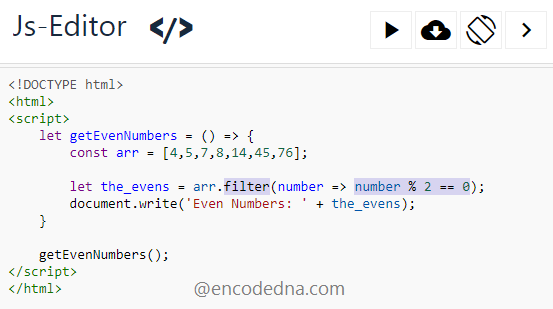﻿ How to find Even Numbers in an Array using JavaScript

# How to find Even Numbers in an Array using JavaScript

You can loop through each number in an array and using a formula get the even numbers. However, there’s another method, which is more efficient than the for loop or the forEach() method, it is the JavaScript array filter() method.We’ll see all the three methods here in this post to find even numbers in an array. I have explained each example using ES6 features also.

## Find all Even Numbers in an Array using for loop

Using for loop, you can iterate through each item (numbers in our case) in the array and find the even numbers. For example,

```<script>
function getEvenNumbers() {
var arr = [1, 2, 3, 4, 5, 6];

for (var i = 0; i < arr.length; i++) {
if (arr[i] % 2 === 0) {
document.writeln(arr[i] + "<br />");
}
}
}

getEvenNumbers();
</script>```

Using ES6 features, the script should be,

```<script>
let getEvenNumbers = () => {
const arr = [1, 2, 3, 4, 5, 6];

for (num of arr){
if (num % 2 === 0) {       // 0 for even numbers and 1 for odd numbers.
document.writeln(num + "<br />");
}
}
}

getEvenNumbers();
</script>```

Note: The formula to get all the odd numbers from the array is,

if (num % 2 === 1) { ... } // To get odd numbers.

You may also like: How to filter out only numbers in an Array using Pure JavaScript

## Get Even Numbers using array.forEach() Method

The JavaScript .forEach() method is more efficient than the traditional for loop method, to iterate an array. The formula to get even numbers from the array is similar to similar to the one you saw in the first method above. The technique is slightly different.

```<script>
function getEvenNumbers(num) {
if (num % 2 === 0) {
document.writeln(num + "<br />");
}
}

var arr = [1, 2, 3, 4, 5, 6];
arr.forEach(getEvenNumbers);
</script>
</html>```

Using ES6 features,

```let getEvenNumbers = (num) => {
if (num % 2 === 0) {
document.writeln(num + "<br />");
}
}
const arr = [1, 2, 3, 4, 5, 6];
arr.forEach(getEvenNumbers);```

You may also like: 👉 How to replace Hyphen in a string with a Space using plain JavaScript

## Find all Even numbers in an Array using .filter() method

The .filter() method is more efficient (Why? read the conclusion below) than both the methods that I have shown in the above examples.

```<script>
function getEvenNumbers() {
var arr = [4,5,7,8,14,45,76];

var evens = arr.filter(number => number % 2 == 0);
document.write('Even Numbers: ' + evens);
}

getEvenNumbers();
</script>
</html>```

the above script using ES6 features...

```let getEvenNumbers = () => {
let arr = [4,5,7,8,14,45,76];

let the_evens = arr.filter(number => number % 2 == 0);
document.write('Even Numbers: ' + the_evens);
}

getEvenNumbers();```

The Array.filter() method returns a new array of items that has to pass a test function (the value, which can be a number or a string, has to pass a condition). Therefore, if the condition returns true, the item (a number, in our case) is added to the new array.

Conclusion

Now, if you compare the .filter() method with the for loop and forEach(), the .filter() is a one line solution, where the focus is on the test function (the logic here is: number => number % 2 == 0), no need to run a loop explicitly. The method will take care of everything else.

And hey... the formula to get all the odd numbers from the array is,

if (num % 2 === 1) { ... }   // To get odd numbers.

Use 0 for even numbers and 1 for odd numbers.

Well, that’s it. Thanks for reading.